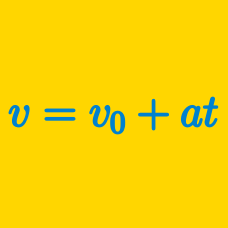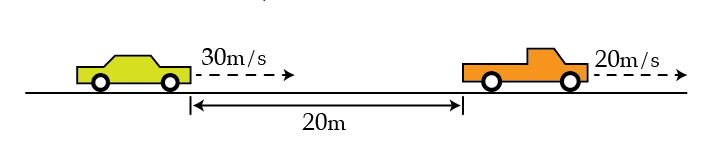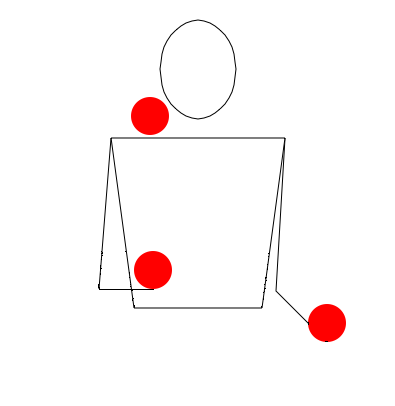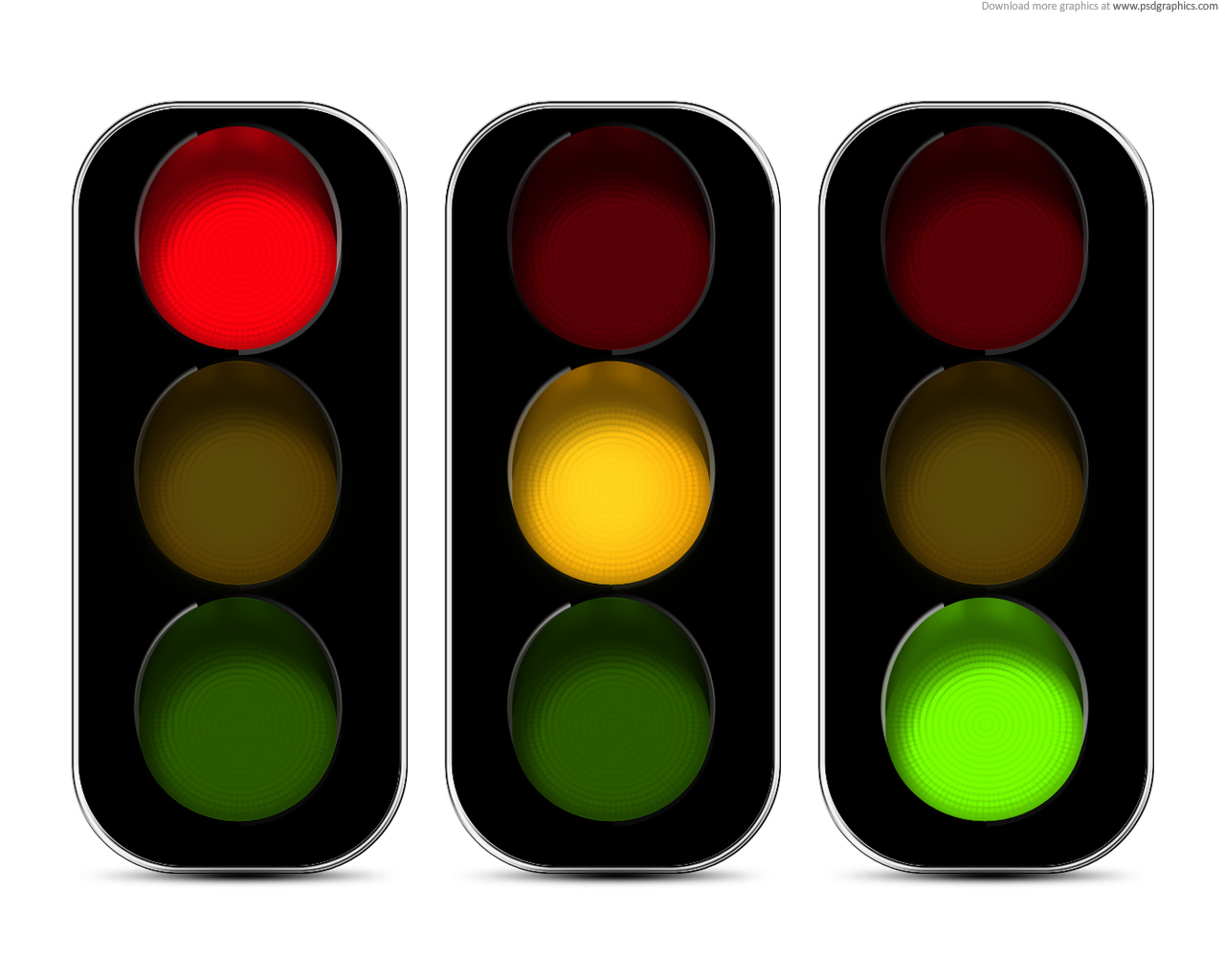Classical Mechanics

1D Kinematics: Level 2-3 Challenges

You are driving a car at $30\text{ m/s}$ when a bad driver traveling at a constant speed of $20\text{ m/s}$ swerves into your lane $20$ meters ahead. As soon as you see the car in front of you, you begin to brake. What is the smallest deceleration needed, in $\text{m/s}^2,$ to avoid rear-ending the car? You may assume your acceleration is constant.A juggler is performing in a circus.

Assume that the juggler is a master and throws each ball up to the same height and throws each ball with the same velocity. He throws each ball when the previous ball has reached the maximum height.

If he throws $n=2$ balls every second, then calculate the maximum height that each ball reaches. Take $g = 10 \text{ m/s}^2$.

Image Credit: Wikimedia 3-ball cascade movie

A Policeman saw a Thief at a distance of $200$ metres. The Policeman and the Thief started running at the same time. If the Policeman runs at a speed of $4\frac{1}{6}$ metres per second and the Thief at a speed of $3 \frac{1}{3}$ metres per second, after what time (in seconds) will the Policeman catch the Thief ?

Two balls of equal masses are thrown vertically upward at an interval of 2 seconds with the same initial speed of 39.2 m/s. At what height will they collide?

Use $g = 9.8 \si{\meter/\second}^2$.Alice is VERY late for school and her dad is giving her a ride. He's driving at a speed of $40 \text{ m/s}$ when the stoplight in front of him turns yellow. At this point, Alice's dad has to make a choice between two options:

1. He could speed up and try to make it past the stoplight before it turns red.

2. He could slow down and bring the car to a stop before he reaches the light and wait for the light to turn green again.

Unfortunately, the distance between the car and the stoplight falls within a particular range such that neither option will work, and he will end up going through the red light no matter what. He can't speed up fast enough to make it through the light, and he's traveling too fast to stop the car before it passes through the red light. If this range can be written as $(a,b)$, where $a$ is the lower bound and $b$ is the upper bound, what is $a+b$?

Details and Assumptions:

• The car both accelerates and decelerates at $8 \text{ m/s}^2$.

• The stoplight is yellow for only 2 seconds before it turns red.

• Assume that Alice's Dad's reaction time is immediate and he either accelerates or decelerates the moment the light turns yellow.

• Disregard friction and air resistance.

×